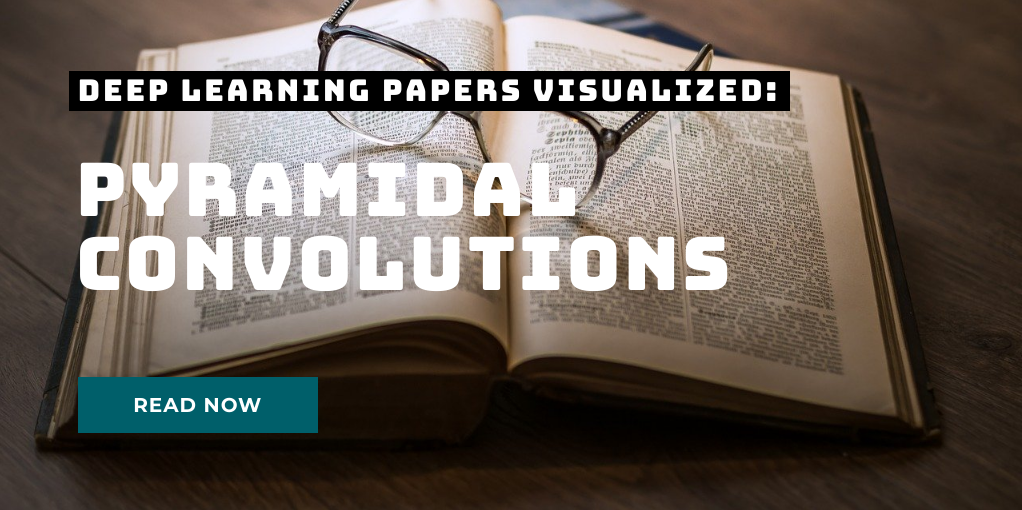# Refactoring machine learning code - namedtuple

Instead of using sometimes confusing indexing in your code, use a namedtuple instead. It’s backwards compatible, so you can still use the index, but you can make your code much more readable. This is especially helpful when you transform between PIL and numpy based code, where PIL uses a column, row notation while numpy uses a row, column notation. Let’s consider this piece of code where we want to get the pixel locations of several points which are in the numpy format:

# Refactoring machine learning code - einops

Einops is a really great library to improve your machine learning code. It supports Numpy, PyTorch, Tensorflow and many more machine learning libraries. It helps to give more semantic meaning to your code and can also save you a lot of headaches when transforming data. As a primer let’s look at a typical use-case in machine learning where you have a bunch of data and you want to reshape it, so some dimensions are merged together like this:

# Refactoring machine learning code - comments as code

I find that in the field of data science and machine learning some coding principles that are standard in traditional software engineering sometimes are lacking. One such principle is to strive to rather specify everything that is possible in code rather than as comments. Why does it make sense to do that? Comments often don’t age well. You write them in the context of the current code, but then over time as the code gets changed and readapted to other use cases, the context changes.

# Depthwise Separable Convolutions in PyTorch

In many neural network architectures like MobileNets, depthwise separable convolutions are used instead of regular convolutions. They have been shown to yield similar performance while being much more efficient in terms of using much less parameters and less floating point operations (FLOPs). Today, we will take a look at the difference of depthwise separable convolutions to standard convolutions and will analyze where the efficiency comes from. Short recap: standard convolution In standard convolutions, we are analyzing an input map of height H and width W comprised of C channels.

# Pyramidal Convolution: Rethinking Convolutional Neural Networks for Visual RecognitionToday’s paper: Pyramidal Convolution by Duta et al. This is the third paper of the new series Deep Learning Papers visualized and it’s about using convolutions in a pyramidal style to capture information of different magnifications from an image. The authors show how a pyramidal convolution can be constructed and apply it to several problems in the visual domain. What’s really interesting is that the number of parameters can be kept the same while performance tends to improve.
Follow me on twitter!